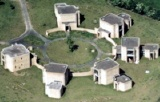# Courses on PDE/Fluids Mechanics

### 13-16 May 2019

A programme of four mini-courses on the Euler equations and vortex filaments.

Organisers: Ben Pooley, Jose Rodrigo

#### Courses:

##### The mathematics of the Onsager's conjecture -- Sara Daneri (l'Aquila)

In 1949, L. Onsager formulated the following conjecture: all solutions of the incompressible Euler equations which are H\"older continuous with exponent bigger than $1/3$ conserve the total kinetic energy, while there are solutions which dissipate the total kinetic energy in the space of H\"older continuous functions with exponent smaller than $1/3$. The conjecture was formulated in accordance with Kolmogorov' s '41 theory of turbulence. The first part of the conjecture, trivial for $C^1$ solutions, was proved by Constantin, E and Titi in '94, using mollification and commutator estimates. The second part of the conjecture challenged the mathematical community for a long time. The first non-conservative solutions of the incompressible Euler equations where found by Scheffer and Shnirelman in the '90s. Then in 2007 De Lellis and Szekelyhidi discovered that the method of convex integration, used for the first time by Nash in '54 to prove the existence of infinitely many $C^1$ isometric embeddings of $n$-dimensional Riemannian manifolds in $\mathbb{R}^{n+2}$, could be developed to produce infinitely many bounded weak solutions with non-constant energy. The regularity of such dissipative solutions was improved up to the critical exponents $1/3$ due to the contribution of various authors, among which De Lellis, Szekelyhidi, Isett, Buckmaster and myself, culminating into the proof of the second part of the Onsager's conjecture by Isett in 2016. In this series of lectures, after giving an overview of the results which led to the proof of the conjecture, I will explain the main ideas of the proof, underlying the technical issues and the strategies to overcome it.

##### An Introduction to Beltrami fields -- Daniel Peralta-Salas (ICMAT)

A divergence-free vector field in Euclidean space whose curl is proportional to the field itself (through a not necessarily constant factor) is called a Beltrami field. From the physical viewpoint, Beltrami fields are a particularly relevant class of stationary solutions to the 3D Euler equations. The goal of this course is to introduce a selected kit of tools to study Beltrami fields, which are instrumental to analyze the topology of the vortex lines and tubes and to establish the existence of vortex reconnections in Navier-Stokes. The first tool that I will present is a Cauchy-Kowalewsky theorem for the curl operator. Then I will explain the local-global flexibility of the Beltrami equation by proving a Runge-type approximation theorem. Finally, I will introduce an inverse localization technique that allows one to transplant Beltrami fields in compact sets of Euclidean space into Beltrami fields on the torus.

##### Vortex filaments in the Euler equations -- Robert Jerrard (Toronto)

An old and prominent conjecture predicts the existence of solutions of the incompressible Euler equations, describing ideal fluid flow, for which the vorticity is concentrated in thin filaments whose evolution is governed by a particular geometric evolution equation. This "vortex filament equation", also known as the binormal curvature flow or the local induction approximation, itself has a very rich mathematical structure. This course will introduce a measure theoretic approach to the study of the binormal curvature flow, and it will discuss implications of this approach for the conjecture about vortex filaments in the Euler equation.

##### The vortex filament equation, the Talbot effect and non-circular jets -- Luis Vega (UPV/EHU)
1.- Vortex Filament Equation: the localized induction approximation of Biot-Savart Integral and Hasimoto transformation.
2.- Self-similar solutions: characterization and their connection with the case of regular polygons. The turbulence of non-circular jets. Non-linear Talbot effect

3.- Skew polygonal lines: self-similar solutions have finite energy. Formation of singularities and continuation of the solutions beyond the blow up time.

#### Analysis Seminar (B3.02 4pm Thursday 16/05)

On the Type I blow-up for the incompressible Euler equations -- Dongho Chae(Chung-Ang University, Korea)

In this talk we discuss the Type I blow up and the related problems in the 3D Euler equations. We say a solution $v$ to the Euler equations satisfies Type I condition at possible blow up time $T_*$ if $\lim\sup_{t\nearrow T_*} (T_*-t) \|\nabla v(t)\|_{L^\infty} <+\infty$. The scenario of Type I blow up is a natural generalization of the self-similar(or discretely self-similar) blow up. We present some recent progresses of our study regarding this. We first localize previous result that small Type I blow up" is absent. After that we show that the atomic concentration of energy is excluded under the Type I condition. This result, in particular, solves the problem of removing discretely self-similar blow up in the energy conserving scale, since one point energy concentration is necessarily accompanied with such blow up. We also localize the Beale-Kato-Majda type blow up criterion. Using similar local blow up criterion for the 2D Boussinesq equations, we can show that Type I and some of Type II blow up in a region off the axis can be excluded in the axisymmetric Euler equations. These are joint works with J. Wolf.

#### Schedule:

Time\Date
(Lecture Room)
Monday 13/05
(MS.04)
Tuesday 14/05
(B3.03)
Wednesday 15/05
(B3.03)
Thursday 16/05
(B3.03)
09:30-11:00   Daneri Jerrard Peralta-Salas
11:00-11:30 Coffee
11:30-12:30 Peralta-Salas Daneri Jerrard
12:30-14:00 Lunch
14:00-15:30 Daneri Jerrard Peralta-Salas
15:30-16:00 Coffee
16:00-17:00 Vega Vega Vega Seminar (B3.02)
17:00-17:30
17:30 Wine ReceptionInternet Access at Warwick:
Where possible, visitors should obtain an EDUROAM account from their own university to enable internet access whilst at Warwick.
If you need WiFi whilst at Warwick, click here for instructions (upon arrival at Warwick)
Registration:
You can register for any of the symposia or workshops online. To see which registrations are currently open and to submit a registration, please click hereLink opens in a new window.
Contact:
Mathematics Research Centre
Zeeman Building
University of Warwick
Coventry CV4 7AL - UK
E-mail:
MRC@warwick.ac.uk